# 1486 Mysterious Cat

### Today’s Puzzle:

If a cat has nine lives, how many lives do seven cats have? Where do the numbers 9 and 7 belong in this puzzle? Where do all the other numbers from 1 to 10 belong?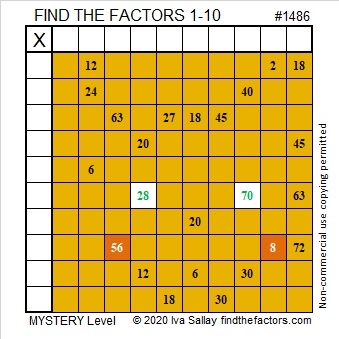### Factors of 1486:

• 1486 is a composite number.
• Prime factorization: 1486 = 2 × 743
• 1486 has no exponents greater than 1 in its prime factorization, so √1486 cannot be simplified.
• The exponents in the prime factorization are 1, and 1. Adding one to each exponent and multiplying we get (1 + 1)(1 + 1) = 2 × 2 = 4. Therefore 1486 has exactly 4 factors.
• The factors of 1486 are outlined with their factor pair partners in the graphic below.### More about the Number 1486:

1486 is not the sum of two squares or the difference of two squares, but it is in a Pythagorean triple:
1486-552048-552050, calculated from 2(743)(1), 743² – 1², 743² + 1²

# 1485 Sticky Lollipop Mystery

### Today’s Puzzle:

Lollipops can be very sticky. Will the logic needed for this puzzle be a sticky mess, or will you be able to lick it? That’s the mystery. Good luck!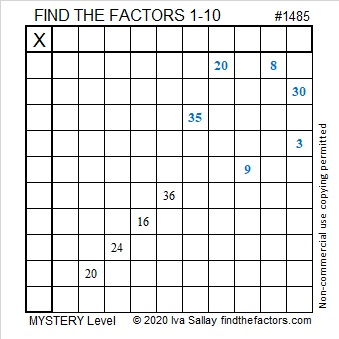### Factors of 1485:

• 1485 is a composite number.
• Prime factorization: 1485 = 3 × 3 × 3 × 5 × 11, which can be written 1485 = 3³ × 5 × 11
• 1485 has at least one exponent greater than 1 in its prime factorization so √1485 can be simplified. Taking the factor pair from the factor pair table below with the largest square number factor, we get √1485 = (√9)(√165) = 3√165
• The exponents in the prime factorization are 3,1 and 1. Adding one to each exponent and multiplying we get (3 + 1)(1 + 1) (1 + 1) = 4 × 2 × 2 = 16. Therefore 1485 has exactly 16 factors.
• The factors of 1485 are outlined with their factor pair partners in the graphic below.### More about the Number 1485:

Did you notice: (27)(55) = 1485? That means that
(54)(55)/2 = 1485 so 1485 is the 54th triangular number.

1485 is the hypotenuse of a Pythagorean triple:
891-1188-1485 which is (3-4-5) times 297.

Of the ten numbers from 1480 to 1489, four are prime numbers and have exactly 2 factors. Three of the ten numbers have exactly 16 factors, namely 1480, 1482, and 1485. No smaller set of three numbers with sixteen factors are as close together as these three are!

# 1484 A Popsicle for a Hot Summer Day

### Today’s Puzzle:

It can be tricky to eat a melting popsicle on a hot summer day. Likewise, it can be tricky to solve a level 6 puzzle even if it looks like a popsicle!  Making it was my granddaughter’s idea. We hope you enjoy it!### Factors of 1484:

If you know that 7 x 12 is 84, it isn’t hard to recognize that 1484 is divisible by 7.

• 1484 is a composite number.
• Prime factorization: 1484 = 2 × 2 × 7 × 53, which can be written 1484 = 2² × 7 × 53
• 1484 has at least one exponent greater than 1 in its prime factorization so √1484 can be simplified. Taking the factor pair from the factor pair table below with the largest square number factor, we get √1484 = (√4)(√371) = 2√371
• The exponents in the prime factorization are 2, 1, and 1. Adding one to each exponent and multiplying we get (2 + 1)(1 + 1)(1 + 1) = 3 × 2 × 2 = 12. Therefore 1484 has exactly 12 factors.
• The factors of 1484 are outlined with their factor pair partners in the graphic below.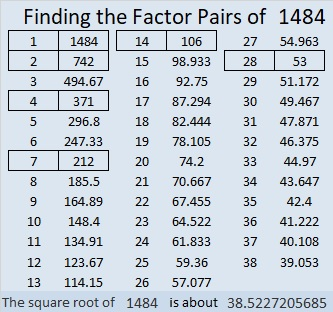### Other facts about the Number 1484:

1484 is the difference of two squares in two different ways:
372² – 370² = 1484
60² – 46² = 1484

784-1260-1484 which is 28 times (28-45-53)

# 1483 and Level 5

### Today’s Puzzle:

Some of the clues in the same row or column in this puzzle have more than one common factor. In each case, will you make the logical choice to find the puzzle’s unique solution?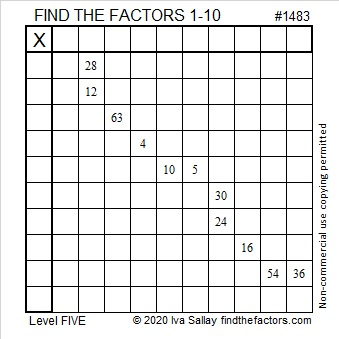### Factors of 1483:

• 1483 is a prime number.
• Prime factorization: 1483 is prime.
• 1483 has no exponents greater than 1 in its prime factorization, so √1483 cannot be simplified.
• The exponent in the prime factorization is 1. Adding one to that exponent we get (1 + 1) = 2. Therefore 1483 has exactly 2 factors.
• The factors of 1483 are outlined with their factor pair partners in the graphic below.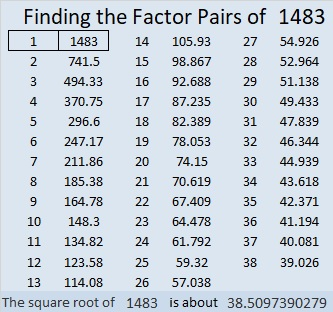How do we know that 1483 is a prime number? If 1483 were not a prime number, then it would be divisible by at least one prime number less than or equal to √1483. Since 1483 cannot be divided evenly by 2, 3, 5, 7, 11, 13, 17, 19, 23, 29 31, or 37, we know that 1483 is a prime number.

### More about the Number 1483:

1483 is the difference of two squares:
742² – 741² = 1483

The first five prime decades are listed below. 1483 is the second prime number in the fifth prime decade: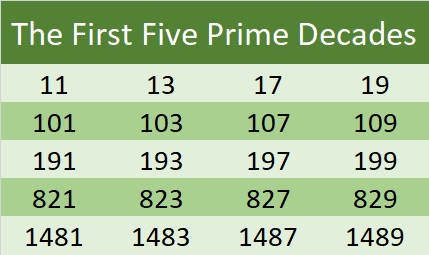# 1480 and Level 3

### Today’s Puzzle:

This is a level 3 puzzle. Start with the common factor of 21 and 14, then fill in the cells in the first column in order from top to bottom as well as the factors in the top row until you have placed all of the factors from 1 to 10 in both of those places.### Factors of 1480:

80 is divisible by 8 and 4 is even, so 1480 is divisible by 8. Here’s a little more about its factors:

• 1480 is a composite number.
• Prime factorization: 1480 = 2 × 2 × 2 × 5 × 37, which can be written 1480 = 2³ × 5 × 37
• 1480 has at least one exponent greater than 1 in its prime factorization so √1480 can be simplified. Taking the factor pair from the factor pair table below with the largest square number factor, we get √1480 = (√4)(√370) = 2√370
• The exponents in the prime factorization are 3,1 and 1. Adding one to each exponent and multiplying we get (3 + 1)(1 + 1) (1 + 1) = 4 × 2 × 2 = 16. Therefore 1480 has exactly 16 factors.
• The factors of 1480 are outlined with their factor pair partners in the graphic below.### Something else about the number 1480:

1480 is the hypotenuse of FOUR Pythagorean triples:
456-1408-1480 which is 8 times (57-176-185)
480-1400-1480 which is (12-35-37) times 40
832-1224-1480 which is 8 times (104-153-185)
888-1184-1480 which is (3-4-5) times 296

# 1479 and Level 2

### Today’s Puzzle:

Can you find the factors from 1 to 10 that will work with the clues in this puzzle to make a multiplication table?### Factors of 1479:

• 1479 is a composite number.
• Prime factorization: 1479 = 3 × 17 × 29
• 1479 has no exponents greater than 1 in its prime factorization, so √1479 cannot be simplified.
• The exponents in the prime factorization are 1, 1, and 1. Adding one to each exponent and multiplying we get (1 + 1)(1 + 1)(1 + 1) = 2 × 2 × 2 = 8. Therefore 1479 has exactly 8 factors.
• The factors of 1479 are outlined with their factor pair partners in the graphic below.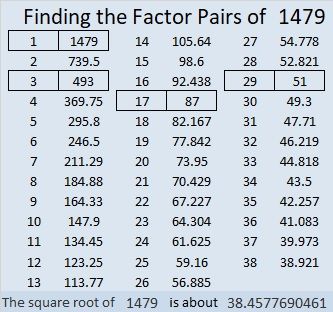### Another Fact about the Number 1479:

1479 is the hypotenuse of FOUR Pythagorean triples:
396-1425-1479 which is 3 times (132-475-493)
465-1404-1479 which is 3 times (155-468-493)
696-1305-1479 which is (8-15-17) times 87
1020-1071-1479 which is (20-21-29) times 51

# 1478 and Level 1

### Today’s Puzzle:

If you can divide a few even numbers in half, then you can find all the factors needed to solve this puzzle. Then you can complete the multiplication table that the puzzle makes. Go for it!### Factors of 1478:

• 1478 is a composite number.
• Prime factorization: 1478 = 2 × 739
• 1478 has no exponents greater than 1 in its prime factorization, so √1478 cannot be simplified.
• The exponents in the prime factorization are 1, and 1. Adding one to each exponent and multiplying we get (1 + 1)(1 + 1) = 2 × 2 = 4. Therefore 1478 has exactly 4 factors.
• The factors of 1478 are outlined with their factor pair partners in the graphic below.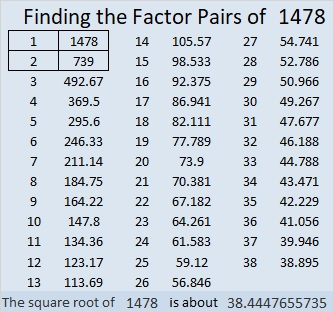### A little more about the number 1478:

1478 is not the sum or the difference of two squares, but it is the short leg in a Pythagorean triple:
(1478-546120-546122) calculated from 2(739)(1), 739² – 1², 739² + 1².

# 1477 Challenge

### Today’s Puzzle:

It’s been a few days since I’ve last looked at this puzzle, but I was still able to solve it in 17 minutes. Can you beat my time?Print the puzzles or type the solution in this excel file: 12 Factors 1468-1477

### Factors of 1477:

• 1477 is a composite number.
• Prime factorization: 1477 = 7 × 211
• 1477 has no exponents greater than 1 in its prime factorization, so √1477 cannot be simplified.
• The exponents in the prime factorization are 1, and 1. Adding one to each exponent and multiplying we get (1 + 1)(1 + 1) = 2 × 2 = 4. Therefore 1477 has exactly 4 factors.
• The factors of 1477 are outlined with their factor pair partners in the graphic below.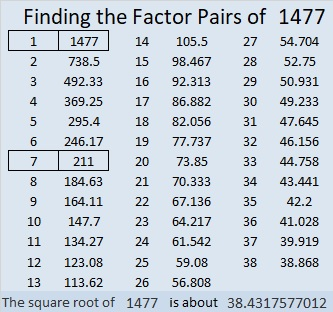### Another Fact about the Number 1477:

1477 is the difference of two squares in two different ways:
739² – 738² = 1477
109² – 102² = 1477

# 1476 Mystery

### Today’s Puzzle:

To solve this mystery puzzle, first, gather some facts. I mean, common factors:
Common Factors of 6, 3, 12, and 9 are 1 and 3.
For 20 and 10 we have 2, 5, and 10.
And for 20 and 60, we can only use 5 or 10 because common factor, 20, is too big for a 1 to 12 puzzle.

Which common factors “Done it”? Don’t jump to conclusions. Remember, a good detective will use the facts and logic to figure out the mystery. Good luck!### Factors of 1476:

• 1476 is a composite number.
• Prime factorization: 1476 = 2 × 2 × 3 × 3 × 41, which can be written 1476 = 2² × 3² × 41
• 1476 has at least one exponent greater than 1 in its prime factorization so √1476 can be simplified. Taking the factor pair from the factor pair table below with the largest square number factor, we get √1476 = (√36)(√41) = 6√41
• The exponents in the prime factorization are 2, 2, and 1. Adding one to each exponent and multiplying we get (2 + 1)(2 + 1)(1 + 1) = 3 × 3 × 2 = 18. Therefore 1476 has exactly 18 factors.
• The factors of 1476 are outlined with their factor pair partners in the graphic below.Simplifying √1476 doesn’t have to be a mystery! Here’s one strategy to do it: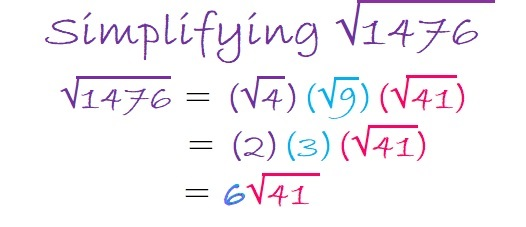### More Facts about the number 1476:

1476 is the sum of two squares:
30² + 24² = 1476

1476 is the hypotenuse of a Pythagorean triple:
324-1440-1476 which is 36 times (9-40-41),
and can also be calculated from 30² – 24², 2(30)(24), 30² + 24².

# 1475 The Logic Needed to Solve This Mystery

### Today’s Puzzle:

Solving this puzzle will be as easy as 1-2-3 if you begin by asking yourself which clues must use the 1’s, the 2’s, and the 3’s, but not necessarily in that order. At the same time, you can eliminate two common factors of 48 and 72, and finishing the puzzle should then become fairly routine.### Factors of 1475:

• 1475 is a composite number.
• Prime factorization: 1475 = 5 × 5 × 59, which can be written 1475 = 5² × 59
• 1475 has at least one exponent greater than 1 in its prime factorization so √1475 can be simplified. Taking the factor pair from the factor pair table below with the largest square number factor, we get √1475 = (√25)(√59) = 5√59
• The exponents in the prime factorization are 2 and 1. Adding one to each exponent and multiplying we get (2 + 1)(1 + 1) = 3 × 2 = 6. Therefore 1475 has exactly 6 factors.
• The factors of 1475 are outlined with their factor pair partners in the graphic below.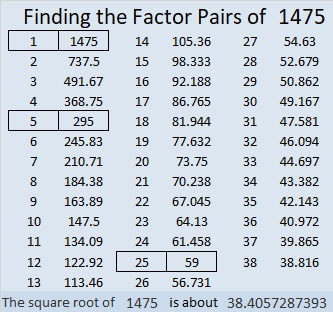### Another Fact about the Number 1475:

1475 is the hypotenuse of two Pythagorean triples:
413-1416-1475 which is (7-24-25) times 59.
885-1180-1475 which is (3-4-5) times 295.